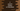# C program to convert Celsius to Fahrenheit## C program to convert Celsius to Fahrenheit :

In this C programming tutorial, we will learn how to convert celsius to fahrenheit using user provided values. We will write one program that will take the value of celsius from the user and print out its fahrenheit value. With this program, you will learn how to take user inputs and how to do mathematical conversions in C.

I will show you two different ways to solve this problem: By directly converting the value and by using a function.

### Celsius to Fahrenheit formula :

Below is the formula used to convert celsius to Fahrenheit :

``Fahrenheit = ((9 * celsius)/5) + 32``

So, using this formula, we can find out fahrenheit if celsius is given.

### C Program :

Below is the complete C program :

``````#include <stdio.h>

int main()
{
float fahrenheit, celsius;

printf("Enter the temperature value in celsius : ");
scanf("%f", &celsius);

fahrenheit = ((9 * celsius)/5) + 32;

printf("Fahrenheit value : %.2f\n", fahrenheit);
}``````
• Here, we are defining two floating point variables fahrenheit and celsius to store the values of fahrenheit and celsius.
• Using printf, we are asking the user to enter the temperature and storing it in celsius variable using scanf.
• Then, we are calculating the value of fahrenheit using the same formula shown above.
• Finally, we are printing the value of fahrenheit using printf.

### Sample Output:

``````Enter the temperature value in celsius : 32
Fahrenheit value : 89.60

Enter the temperature value in celsius : 100
Fahrenheit value : 212.00``````

### Method 2: Using a function:

We can also use one different function to do the conversion and call that function from main:

``````#include <stdio.h>

float celsiusToFahrenheit(float celsius){
return ((9 * celsius)/5) + 32;
}

int main()
{
float fahrenheit, celsius;

printf("Enter the temperature value in celsius : ");
scanf("%f", &celsius);

printf("Fahrenheit value : %.2f\n", celsiusToFahrenheit(celsius));
}``````

In this method, we are doing the same thing. The only difference is that I have added one new function celsiusToFahrenheit to do the conversion. This function takes the value of celsius, calculates the fahrenheit and returns the fahrenheit value.

It will give similar output as the above program.